# Unsupervised learning and clustering algorithms

Last updated on：2 years ago

Unlabelled data is also a key type of input data of machine learning. Usually, we will cluster these data to discover their structures.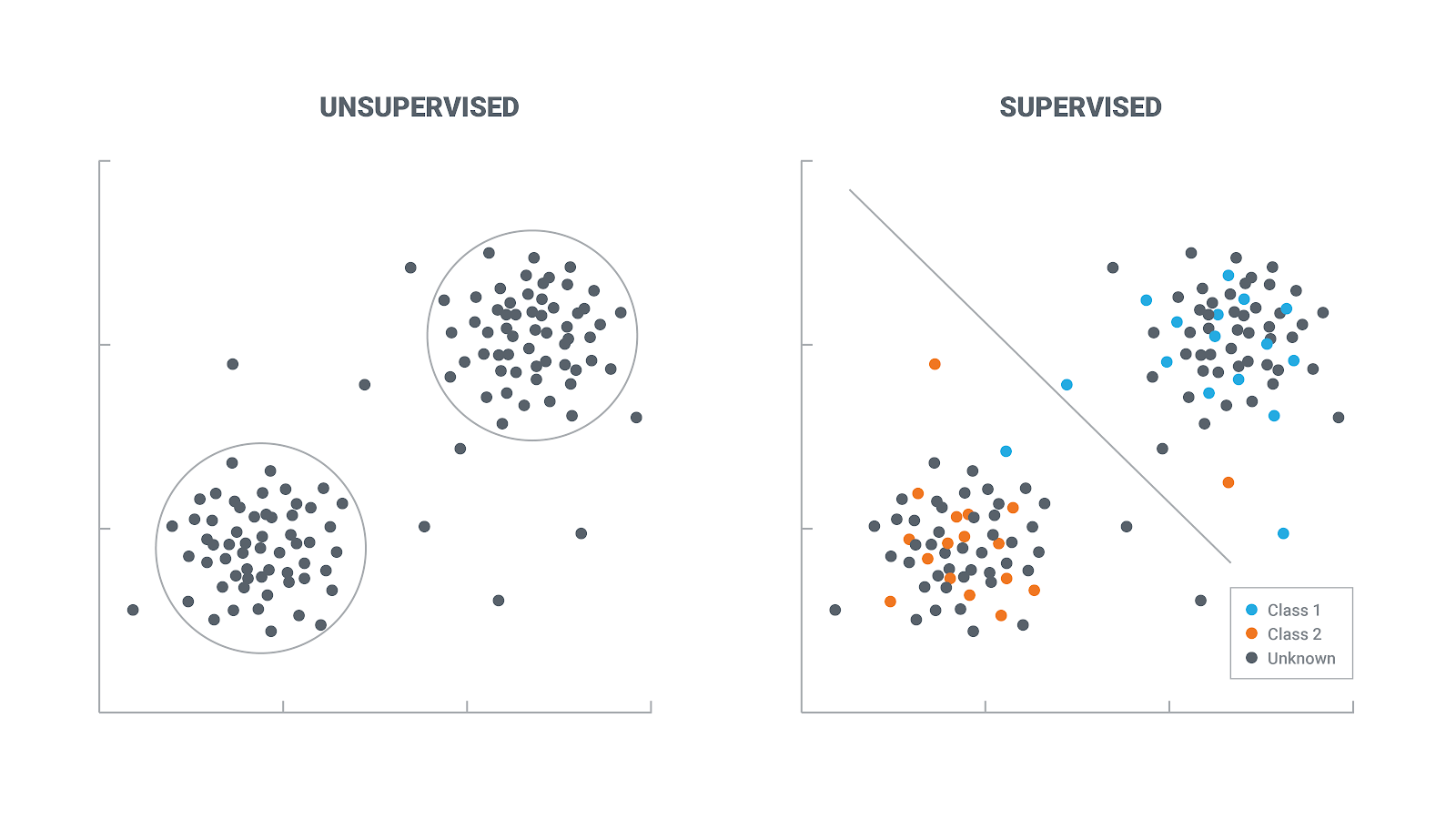In unsupervised learning, you are given an unlabelled dataset and are asked to find “structure” in the data.

# Clustering techniques

Clustering is the unsupervised classification of patterns (observations, data items, or feature vectors) into groups (clusters).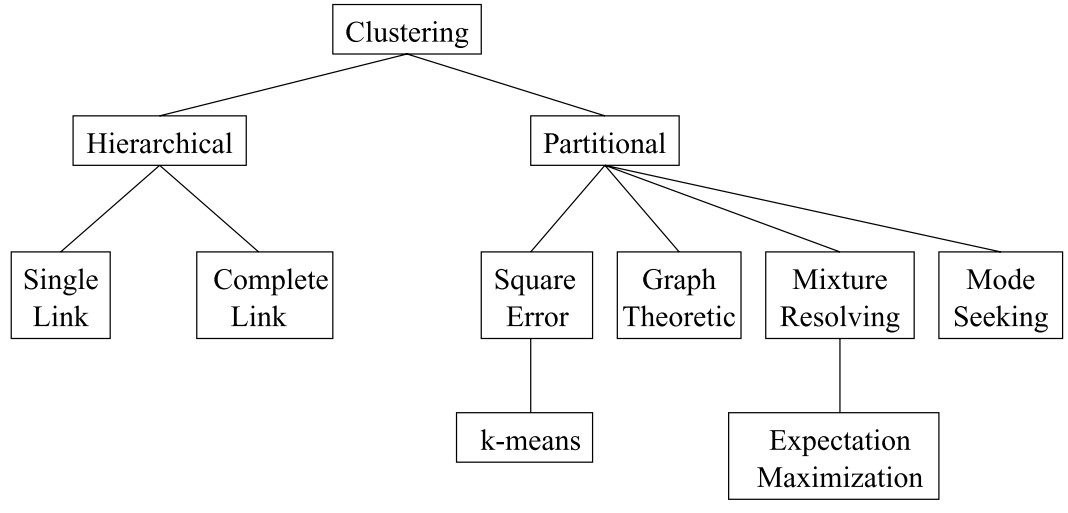Hierarchical clustering algorithms, Mixture-resolving and mode-seeking algorithms, nearest neighbour clustering, Fuzzy clustering, etc.

## Partitional algorithms

### K-means algorithm

Cluster centroid

In mathematics and physics, the centroid or geometric centre of a plane figure is the arithmetic mean position of all the points in the figure.

Steps

• Cluster assignment step - minimize J， wrt $c^{(1)}, c^{(2)}, c^{(k)}$
• Move cluster centroid step - minimize J , with reference to $\mu_1, \mu_2, \mu_k$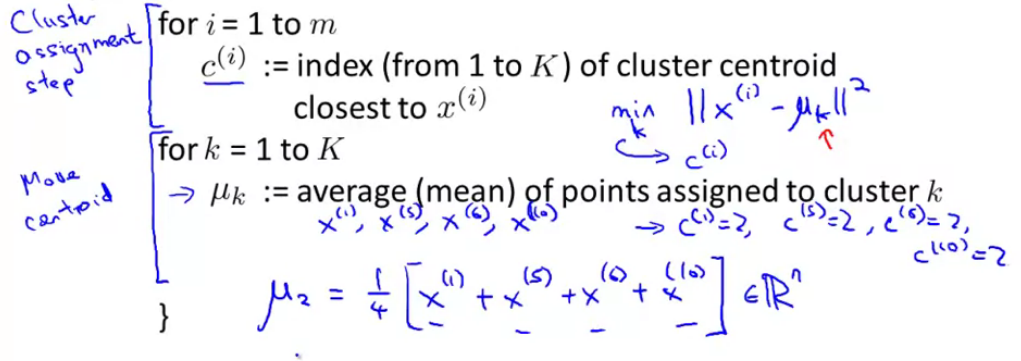Input

• K (number of clusters)
• Unlabelled Training set

#### Randomly initialize K cluster centroid

• Should have $K<m$
• Randomly pick K training examples

• Set $\mu_1, … , \mu_K$ equal to these K examples

Try multiple random initializations. Pick clustering theta gave lowest cost J

#### Optimization objective

Minimize $J(c^{(1)}, …, c^{(m)}, \mu_1, …, \mu_K)$.

#### Choosing the number of clusters

Elbow method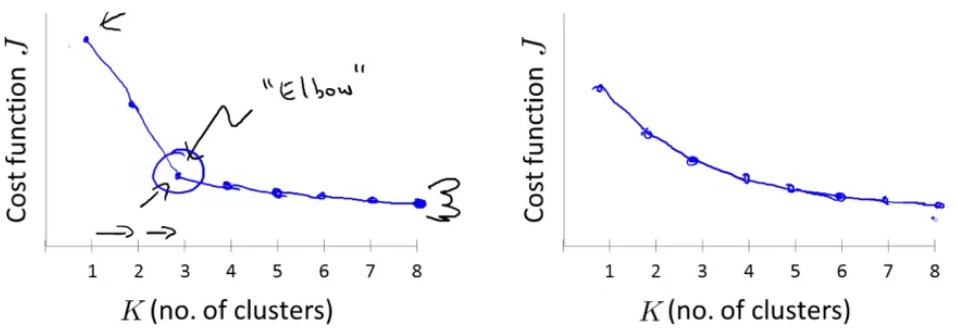### Others

Graph-theoretic clustering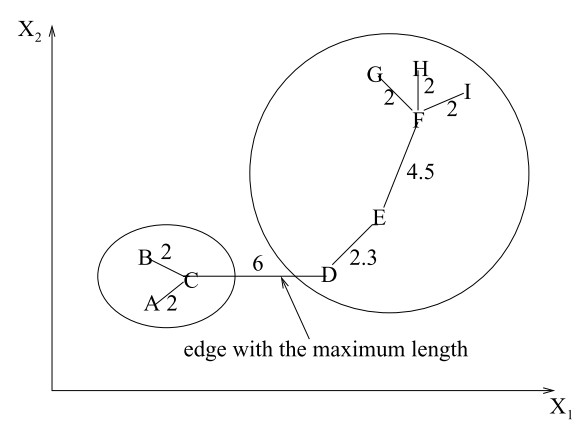# Applications

Image segmentation, object and character recognition, information retrieval, data mining.

# Reference

 Andrew NG, Machine learning

 Centroid

 Jain, A.K., Murty, M.N. and Flynn, P.J., 1999. Data clustering: a review. ACM computing surveys (CSUR), 31(3), pp.264-323.